## What is Euler’s Secret Identity?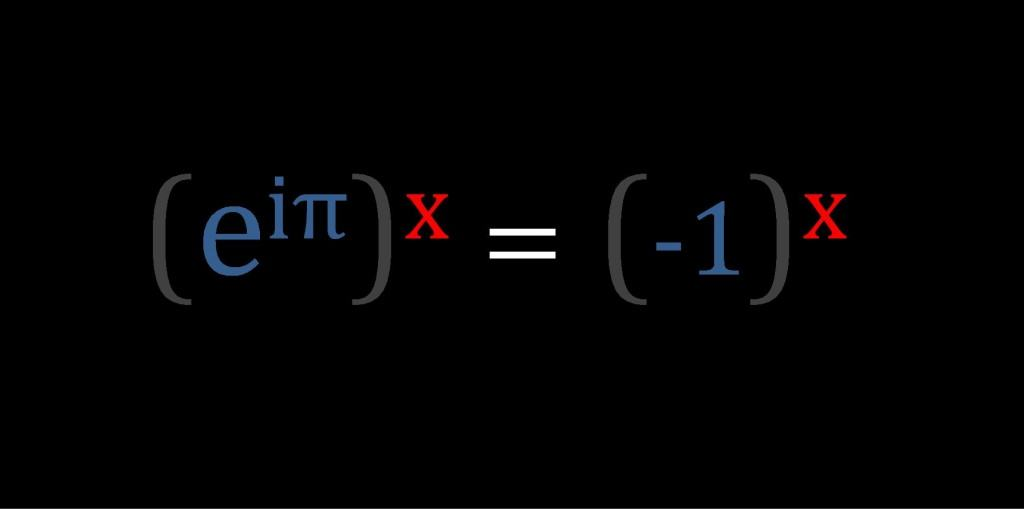Is there some connection between the Mathematics of Euler’s Identity and the Physics of a Photon?

Euler’s Formula (eix = cos(x) + i sin(x) ) is usually thought of as a mathematical description of how a “vector rotates” through an angle in the complex plane; and consequently Euler’s Identity is usually considered simply a rotation through an angle of π radians.  But as long ago as 1581 Galileo had already realized something amazing; “rotation” has a secret identity — he is also an “oscillation”…

Galileo’s startling realization that uniform circular motion and periodic motion were different sides of the same coin, meant that he saw that angles could also be used to describe an oscillation.  And this, it turns out, was a truly brilliant insight!…

So although most people would probably still associate angles with geometry and trigonometry, angles can also be associated with the amount of “energy in an oscillation” –-  which means that a function that defines an amount of  rotation in the complex-plane ( y=(-1+i0))  can also be thought of as a “definable angular frequency” expressed as x% of (-1+i0), or in other words: “ x% of a half-cycle”.

Now, what is really interesting about this idea is that:  the amplitude of all “normalized” oscillations have a magnitude of 0.25 units of measurement, or ¼ of the normalized cycle.  So

• In our formula ( y=(-1+i0)), when we set  x=0.5, we get “a quarter-rotation in the complex plane”

(-1+i0)0.5   =   (0+i1)

• But in setting x=0.5 we are also, in effect, setting an angular frequency of π/2 radians for the equivalent oscillation on the real-line; and so we could also say that (0+i1) is “a quarter-oscillation on the real-line”, which means that

(0+i) is the amplitude of all normalized oscillations

• And this means that

(-1) is the “amplitude of an normalized oscillation squared (i2)”

• And this means that

all frequencies can be defined as some % of (i2)

=======

So, any angular frequency of oscillation, defined in terms of a single half-cycle ( y = (-1)x ), can now also be defined in terms of the normalized amplitude squared  ( y = (i2)x ).  And this, to anyone who has studied even a small amount of physics is strangely reminiscent of the “energy in simple harmonic motion”.

This would seem to suggest that an angular frequency (i2)x  might be equivalent is some way to the energy in an oscillation (½kA2), and that (x) might represent some form of “restoring variable”.

Furthermore since we know that the equation for the energy in a photon of light is

E  =  hf  =  ħω

This further suggests that there might be some form of a connection between (eiπx) and (ħω).  So let’s take a closer look at ( ei )…

We can use Euler’s Formula to define a smallest “unit of angular change”.  Let’s define this unit to have the same magnitude as the value of Planck’s Reduced Constant.

(1 ħ-radian = 1.054571726  ×  10-34 radians)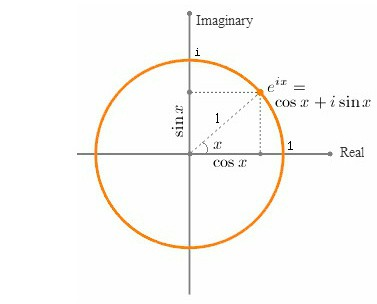eix   =   =   cos(x) +  i sin(x)

ei   =   cos(1) +  i sin(1)

eiħ   =   cos(ħ) +  i sin(ħ)

(-1)ħ   =   (0.99999999 +  iħ)

Given this defined “unit of angular change”, we can now think of every other size of angular change as an integer number(n) of units of (1 +iħ);

(eiħ)n   =   (ei)ħn   =   einħ   =   (1 + iħ)n   =   n ħ-radians   (where n = 1,2,3….)

And when n = Ħ =  9.48252238653324  x  10+33 (i.e. capital-h-bar : the inverse of  ħ) we get an angle of

(eiħ)Ħ   =   (ei)ħĦ   =   ei   =   (1 + iħ)Ħ   =   Ħ ħ-radians =   1 radian

And when n = Ħπ we get an angle of

(eiħ)Ħπ   =   (ei)ħĦπ   =   eiπ   =   (1 + iħ)Ħπ   =   π radians  = (-1+i0)So if (-1)x  is indeed equivalent to  (½kA2) we could now say that since

(-1)x   =   (eiπ)x   =   (1+ iħ) Ħπx

And since

½kA2   =   hf

Therefore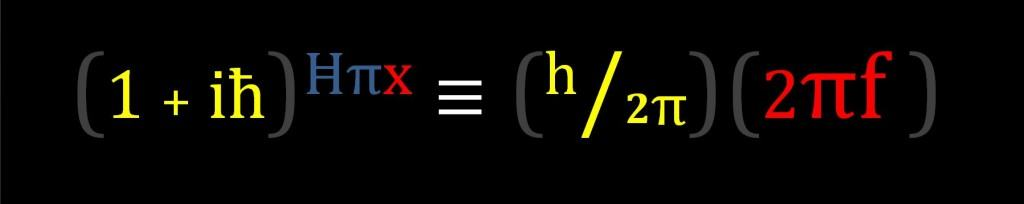So maybe different photon frequencies simply represent different amounts of restoring force in the elastic fabric of space-time.  If so then different frequencies would simply represent different percentages of the ultimate elasticity of space-time.

So what is Euler’s Secret Identity?

Maybe he is the speed of light’s cousin;

“The Angular Speed of Mass” – the fastest frequency of all…

## How Do You Like Them Apples?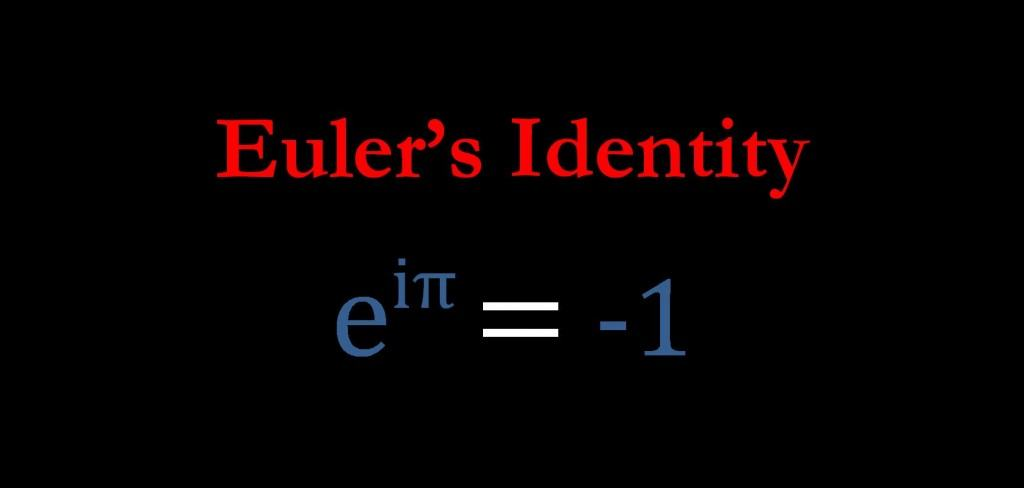Named after the Swiss mathematician Leonhard Euler – Euler’s Identity is often described as an example of deep mathematical beauty.  Some see it almost as proof of the existence of some hidden order of the universe that we can only catch a glimpse of…

This equation is considered amazing because it seems to sets up a relationship between three apparently completely unrelated subjects.

“e” originated from the study of compound interest (and was subsequently found to be the base of the natural logarithms); “π” originated from Geometry and Trigonometry; and i” was “invented” to help with solving equations involving negative numbers.

Compound Interest, Trigonometry, and Imaginary Numbers?…  The question that has intrigued mathematicians and physicists since Euler first published his famous formula in 1748, is “Why in God’s name do these (apparently completely unrelated) areas of mathematics fit together so neatly in this beautiful equation?”

###### Well here’s the answer – Watch!

[Note: What follows is just a quick jump-thru, if you want the in-depth understanding click here]

e  =  Limit as n →  (1 + 1/n)n  =  2.718281828459045……

For practical purposes, “e” is difficult to pin down is simply a consequence of the fact that “e” is a manufactured quantity, defined using “limits to infinity”, and infinity is an idea not a number.

In reality there is no definitive value of “Transcendental” “e” because we can theoretically infinitely add more and more decimal places to the value of “e” by constantly increasing n all the way to infinite (which doesn’t actually exist).

However although the concept of infinity might be vague, “the concept of “e””, on the other hand, is actually all about very finely-grained precision…

The concept and formula for “e” is neat and very precise because the number of steps (n) must be the exact inverse of the tiny step-size (1/n).

In order to discuss the preciseness of “e”, it is better, for the purposes of this discussion, to limit the influence of “infinity” and define “e” with very precise numbers.

To do so, we will use both a very large number and a very small number which are exact inverses of each other.  We will use the symbol Ħ for the very large number, and we will use the symbol ħ for the very small number.

Ħ  =  10,000,000,000,000,000,000,000,000,000,000,000  =  10+34

ħ  =  0.000,000,000,000,000,000,000,000,000,000,000,1  =  10-34

Since ħ = 1/Ħ we can now write a precision equation for “e” as

e = ( 1  +  ħ )Ħ  =  2.718281828459045……

Okay, so now away we go…

e = ( 1  +  ħ )Ħ

e1/Ħ = ( 1  +  ħ )

eħ = ( 1  +  ħ )

======

Set       d  =  eħ  =  ( 1  +  ħ )

======

dxĦ  =  (1 + ħ)xĦ  =  (eħ)xĦ  =  (eħĦ)x  ex

so

d  =  (1 + ħ)1Ħ  (eħ)1Ħ  =  (eħĦ)1  =  e1  =  2.718281828459…

[Ħ micro-steps of (1+ħ) is equivalent to 1 macro-step of 2.71828]

Okay that was for real numbers, now let’s do complex numbers…

(d)i  =  (ex)i  =  cos(x) +  i sin(x)

(d)i  =  ei  =  cos(1) +  i sin(1)

di  =  eħi  =  cos(ħ) +  i sin(ħ)

di  =  (0.99999999… +  iħ)di(xĦ)   =   ( 1 + iħ )xĦ   =   (eiħ)xĦ   =   (eħĦ)ix   =   eix

so

di(1Ħ)   =   ( 1 + iħ )1Ħ   =   (eiħ)1Ħ   =   (eħĦ)i1   =   ei1

[Ħ micro-steps of (1+iħ) is equivalent to 1 macro-step of 1 Radian]

and

di(3.14159…Ħ)  =   ( 1 + iħ )πĦ   =   (eiħ)πĦ   =   (eħĦ)iπ   =   eiπ

[3.14159…Ħ micro-steps of (1+iħ) is equivalent to 1 macro-step of π Radians]

.

di(πĦ)    =    -1.00 + i0.00

⇒     di(πĦ)  =    -1

.

.

How do you like them apples?

So why is any of this important?

It is important because despite what is generally believed, Euler Identity is not telling us something special about “rotations”; it is telling us something special about “oscillations”.

[Remember: this was just a quick jump-thru; for a more detailed explanation click here.]

Euler’s identity is a beautiful example of the “quantized mathematics of change”.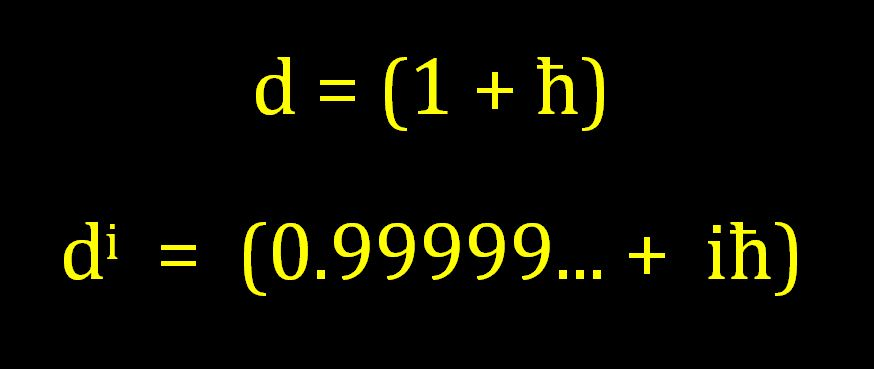This quantization of mathematics allows us to define very precise “coarse” macro step-sizes (both linear and angular) using integer amounts of the quantized micro units d and di.

• d(xĦ) : defines the coarseness of linear growth and decay
• di(xĦ) : defines the coarseness of oscillations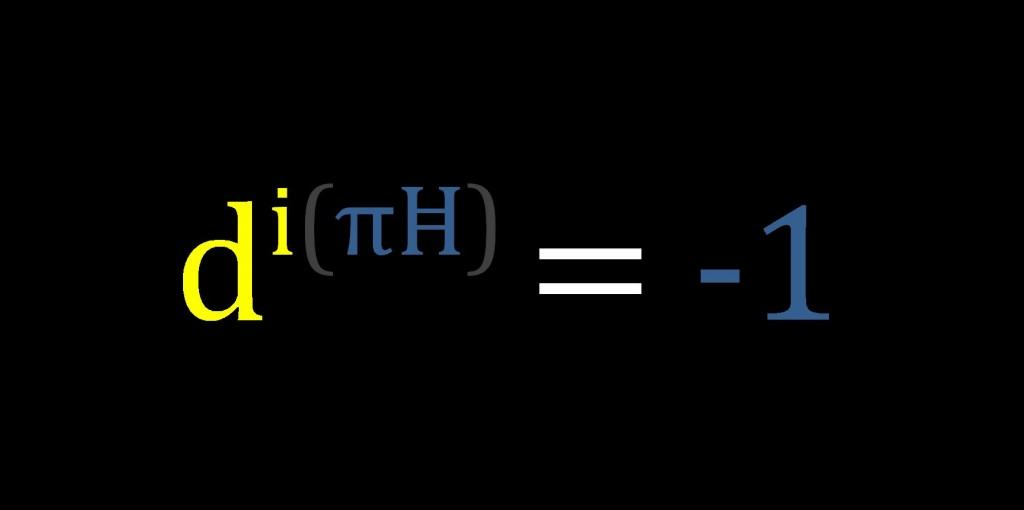[This post is adapted from What is Euler’s Identity?.]